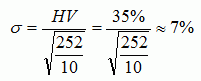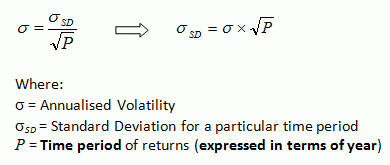I’ve read a few option books.
THANKS... This is probably the most comprehensive "greeks" article/book I’ve read.

Wonderful blog. …..
A wonder wealth of knowledge there. Thanks so much for your kindness in publishing it!

Thank you very much for the most concise and simplest option intro. Highly recommended.

So far, yours is the best blog/site on basic options notes in the web that I have chanced upon.

## Tuesday, August 23, 2011

### Historical Volatility – Part 6: Interpretation

Go back to Part 5: How To Annualise Standard Deviation.

After we know the definition and how to calculate HV, we’ll move on to its interpretation.

Example:
If it is known that the value of HV is 35%. Remember that this HV value is annualised, i.e. for one year.
As mentioned in the earlier post, assuming that price returns are normally distributed, about two-third of the time, an individual return would fall within one standard deviation of the mean, and about 95% of the time, an individual return would fall within two standard deviation of the mean.

That means, we can interpret that in one year, approximately two-thirds of the time, the stock returns would be between minus 35% and plus 35%. Or about 95% of the time, the stock return would be between minus 70% (= 2*35%) and plus 70%.

If the stock price is \$100, in one year, the price would probably be between \$65 and \$135 about two-thirds of the time. Or about 95% of the time, the stock price would be within \$30 to \$170 range.

How about for one month?
Since the annualised HV is 35%, the estimated value of standard deviation for one month will be:That means, in one month, approximately two-thirds of the time, the stock returns would be between minus 10% and plus 10%. Or about 95% of the time, the stock return would be between minus 20% (= 2*10%) and plus 20%.

If the stock price is \$100, in one month, the price would probably be between \$90 and \$110 about two-thirds of the time. Or about 95% of the time, the stock price would be within \$80 to \$120 range.

How about for 10 days?
With the annualised HV of35%, the estimated value of standard deviation for 10 days will be:That means, in 10 days time, approximately two-thirds of the time, the stock returns would be between minus 7% and plus 7%. Or about 95% of the time, the stock return would be between minus 14% (= 2*7%) and plus 14%.

If the stock price is \$100, in 10 days, the price is expected to be between \$93 and \$107 about two-thirds of the time. Or about 95% of the time, the stock price would be within \$86 to \$114 range.

Note:
Some people use the following formula to convert:This formula is actually the same as the above formula.
This formula is based on the one mentioned in Wikipedia, as discussed in the earlier post (Part 5).Continue to Part 7: Comparing HV.

To view the list of all the series on “Historical Volatility”, please refer to: “More Understanding about HISTORICAL VOLATILITY

Other Learning Resources:
* FREE Trading Educational Videos with Special Feature
* FREE Trading Educational Videos from Trading Experts

Related Topics:
* Understanding Implied Volatility (IV)
* Understanding Option Greek
* Understanding Option’s Time Value
* Learning Candlestick Charts
* Options Trading Basic – Part 1
* Options Trading Basic – Part 2